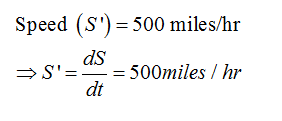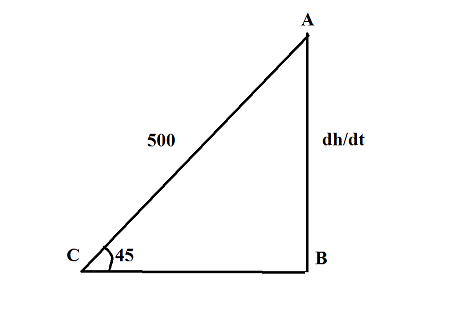# . Betty and Bob take off in an airplane and travel at an angle of 45 degrees to the ground as per figure. If their speed (s’) is 500 miles per hour then at what rate is their altitude, h, increasing at?

Question
1 views

. Betty and Bob take off in an airplane and travel at an angle of 45 degrees to the ground as per figure. If their speed (s’) is 500 miles per hour then at what rate is their altitude, h, increasing at?

check_circle

Step 1

Let dh/dt represent the rate at which altitude is increasing:Step 2

Draw the figure to understand the question clearly:...

### Want to see the full answer?

See Solution

#### Want to see this answer and more?

Solutions are written by subject experts who are available 24/7. Questions are typically answered within 1 hour.*

See Solution
*Response times may vary by subject and question.
Tagged in

### Calculus